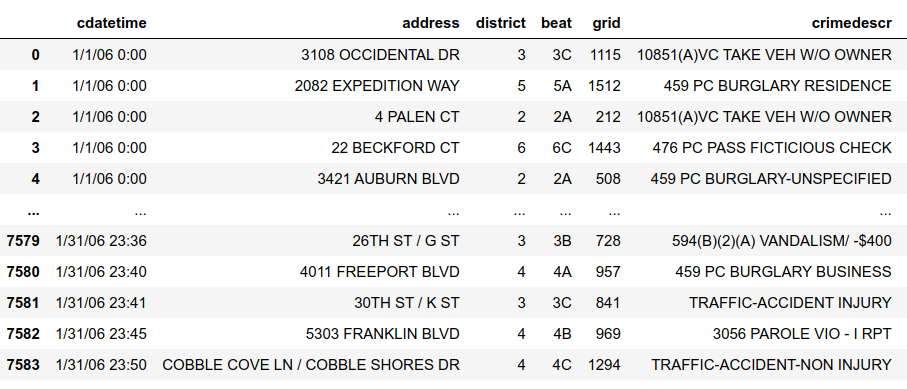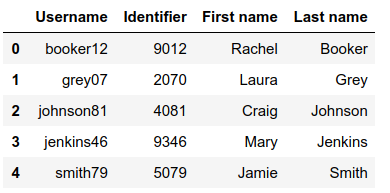# Read multiple CSV files into separate DataFrames in Python

In this article, we will see how to read multiple CSV files into separate DataFrames. For reading only one data frame we can use pd.read_csv() function of pandas. It takes a path as input and returns data frame like

`df = pd.read_csv("file path")`

Let’s have a look at how it works

## Python3

 `# import module` `import` `pandas as pd`   `# read dataset` `df ``=` `pd.read_csv(``"./csv/crime.csv"``)`

Here, crime.csv is the file in the current folder. CSV is the folder that contains the crime.csv file and CSV Reader.ipynb is the file containing the above code.

Output:It is the data frame that is read from the above function. One more file is present in the folder named – username.csv. To read them both and store them in different data frames use the below code

## Python3

 `# import module` `import` `pandas as pd`   `# assign dataset names` `list_of_names ``=` `[``'crime'``,``'username'``]`   `# create empty list` `dataframes_list ``=` `[]`   `# append datasets into the list` `for` `i ``in` `range``(``len``(list_of_names)):` `    ``temp_df ``=` `pd.read_csv(``"./csv/"``+``list_of_names[i]``+``".csv"``)` `    ``dataframes_list.append(temp_df)`

dataframes_list contains all the data frames separately.

dataframes_list:dataframes_list:Here is another approach, now suppose that there are many files, and we don’t know the names and number then, use the below code

## Python3

 `# import modules` `import` `os` `import` `pandas as pd`   `# assign path` `path, dirs, files ``=` `next``(os.walk(``"./csv/"``))` `file_count ``=` `len``(files)` `# create empty list` `dataframes_list ``=` `[]`   `# append datasets to the list ` `for` `i ``in` `range``(file_count):` `    ``temp_df ``=` `pd.read_csv(``"./csv/"``+``files[i])` `    ``dataframes_list.append(temp_df)` `    `  `# display datasets` `for` `dataset ``in` `dataframes_list:` `    ``display(dataset)`

Output:Whether you're preparing for your first job interview or aiming to upskill in this ever-evolving tech landscape, GeeksforGeeks Courses are your key to success. We provide top-quality content at affordable prices, all geared towards accelerating your growth in a time-bound manner. Join the millions we've already empowered, and we're here to do the same for you. Don't miss out - check it out now!

Previous
Next